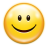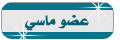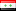منتدى مصر التقني
هل تريد التفاعل مع هذه المساهمة؟ كل ما عليك هو إنشاء حساب جديد ببضع خطوات أو تسجيل الدخول للمتابعة.

# كود لعبة متاهة##كود لعبة متاهة

اهلا وسهلا بكم في منتدى مصر التقني
----------------------------------
كود لعبة متاهة في j2meكود   التالي

الكود:
`import java.util.Random;import java.util.Vector;import javax.microedition.midlet.*;import javax.microedition.lcdui.*;/** * This is the main class of the maze game. * * @author Carol Hamer */public class Maze extends MIDlet implements CommandListener {  //----------------------------------------------------------------  //  game object fields  /**   * The canvas that the maze is drawn on.   */  private MazeCanvas myCanvas;  /**   * The screen that allows the user to alter the size parameters    * of the maze.   */  private SelectScreen mySelectScreen;  //----------------------------------------------------------------  //  command fields  /**   * The button to exit the game.   */  private Command myExitCommand = new Command("Exit", Command.EXIT, 99);  /**   * The command to create a new maze.  (This command may appear in a menu)   */  private Command myNewCommand = new Command("New Maze", Command.SCREEN, 1);  /**   * The command to dismiss an alert error message.  In MIDP 2.0   * an Alert set to Alert.FOREVER automatically has a default    * dismiss command.  This program does not use it in order to    * allow backwards com   */  private Command myAlertDoneCommand = new Command("Done", Command.EXIT, 1);  /**   * The command to go to the screen that allows the user    * to alter the size parameters.  (This command may appear in a menu)   */  private Command myPrefsCommand     = new Command("Size Preferences", Command.SCREEN, 1);  //----------------------------------------------------------------  //  initialization  /**   * Initialize the canvas and the commands.   */  public Maze() {    try {       myCanvas = new MazeCanvas(Display.getDisplay(this));      myCanvas.addCommand(myExitCommand);      myCanvas.addCommand(myNewCommand);      myCanvas.addCommand(myPrefsCommand);      myCanvas.setCommandListener(this);    } catch(Exception e) {      // if there's an error during creation, display it as an alert.      Alert errorAlert = new Alert("error",            e.getMessage(), null, AlertType.ERROR);      errorAlert.setCommandListener(this);      errorAlert.setTimeout(Alert.FOREVER);      errorAlert.addCommand(myAlertDoneCommand);      Display.getDisplay(this).setCurrent(errorAlert);    }  }  //----------------------------------------------------------------  //  implementation of MIDlet  /**   * Start the application.   */  public void startApp() throws MIDletStateChangeException {    if(myCanvas != null) {      myCanvas.start();    }  }    /**   * Clean up.   */  public void destroyApp(boolean unconditional)       throws MIDletStateChangeException {    myCanvas = null;    System.gc();  }  /**   * Does nothing since this program occupies no shared resources    * and little memory.   */  public void pauseApp() {  }  //----------------------------------------------------------------  //  implementation of CommandListener  /*   * Respond to a command issued on the Canvas.   * (reset, exit, or change size prefs).   */  public void commandAction(Command c, Displayable s) {    if(c == myNewCommand) {      myCanvas.newMaze();    } else if(c == myAlertDoneCommand) {      try {          destroyApp(false);          notifyDestroyed();      } catch (MIDletStateChangeException ex) {      }    } else if(c == myPrefsCommand) {      if(mySelectScreen == null) {          mySelectScreen = new SelectScreen(myCanvas);      }      Display.getDisplay(this).setCurrent(mySelectScreen);    } else if(c == myExitCommand) {      try {         destroyApp(false);         notifyDestroyed();      } catch (MIDletStateChangeException ex) {      }    }  }  }/** * This class is the display of the game. *  * @author Carol Hamer */class MazeCanvas extends javax.microedition.lcdui.Canvas {  //---------------------------------------------------------  //   static fields  /**   * color constant   */  public static final int BLACK = 0;  /**   * color constant   */  public static final int WHITE = 0xffffff;  //---------------------------------------------------------  //   instance fields  /**   * a handle to the display.   */  private Display myDisplay;  /**   * The data object that describes the maze configuration.   */  private Grid myGrid;  /**   * Whether or not the currently displayed maze has    * been completed.   */  private boolean myGameOver = false;  /**   * maze dimension: the width of the maze walls.   */  private int mySquareSize;  /**   * maze dimension: the maximum width possible for the maze walls.   */  private int myMaxSquareSize;  /**   * maze dimension: the minimum width possible for the maze walls.   */  private int myMinSquareSize;  /**   * top corner of the display: x-coordiate   */  private int myStartX = 0;  /**   * top corner of the display: y-coordinate   */  private int myStartY = 0;  /**   * how many rows the display is divided into.   */  private int myGridHeight;  /**   * how many columns the display is divided into.   */  private int myGridWidth;  /**   * the maximum number columns the display can be divided into.   */  private int myMaxGridWidth;  /**   * the minimum number columns the display can be divided into.   */  private int myMinGridWidth;  /**   * previous location of the player in the maze: x-coordiate   * (in terms of the coordinates of the maze grid, NOT in terms    * of the coordinate system of the Canvas.)   */  private int myOldX = 1;  /**   * previous location of the player in the maze: y-coordinate   * (in terms of the coordinates of the maze grid, NOT in terms    * of the coordinate system of the Canvas.)   */  private int myOldY = 1;  /**   * current location of the player in the maze: x-coordiate   * (in terms of the coordinates of the maze grid, NOT in terms    * of the coordinate system of the Canvas.)   */  private int myPlayerX = 1;  /**   * current location of the player in the maze: y-coordinate   * (in terms of the coordinates of the maze grid, NOT in terms    * of the coordinate system of the Canvas.)   */  private int myPlayerY = 1;  //-----------------------------------------------------  //    gets / sets  /**   * Changes the width of the maze walls and calculates how    * this change affects the number of rows and columns    * the maze can have.   * @return the number of columns now that the the    *         width of the columns has been updated.   */  int setColWidth(int colWidth) {    if(colWidth < 2) {      mySquareSize = 2;    } else {      mySquareSize = colWidth;    }    myGridWidth = getWidth() / mySquareSize;    if(myGridWidth % 2 == 0) {      myGridWidth -= 1;    }    myGridHeight = getHeight() / mySquareSize;    if(myGridHeight % 2 == 0) {      myGridHeight -= 1;    }    myGrid = null;    return(myGridWidth);  }  /**   * @return the minimum width possible for the maze walls.   */  int getMinColWidth() {    return(myMinSquareSize);  }  /**   * @return the maximum width possible for the maze walls.   */  int getMaxColWidth() {    return(myMaxSquareSize);  }  /**   * @return the maximum number of columns the display can be divided into.   */  int getMaxNumCols() {    return(myMaxGridWidth);  }  /**   * @return the width of the maze walls.   */  int getColWidth() {    return(mySquareSize);  }  /**   * @return the number of maze columns the display is divided into.   */  int getNumCols() {    return(myGridWidth);  }  //-----------------------------------------------------  //    initialization and game state changes  /**   * Constructor performs size calculations.   * @throws Exception if the display size is too    *         small to make a maze.   */  public MazeCanvas(Display d) throws Exception {    myDisplay = d;    // a few calculations to make the right maze     // for the current display.    int width = getWidth();    int height = getHeight();    // tests indicate that 5 is a good default square size,     // but the user can change it...    mySquareSize = 5;    myMinSquareSize = 3;    myMaxGridWidth = width / myMinSquareSize;    if(myMaxGridWidth % 2 == 0) {      myMaxGridWidth -= 1;    }    myGridWidth = width / mySquareSize;    if(myGridWidth % 2 == 0) {      myGridWidth -= 1;    }    myGridHeight = height / mySquareSize;    if(myGridHeight % 2 == 0) {      myGridHeight -= 1;    }    myMinGridWidth = 15;    myMaxSquareSize = width / myMinGridWidth;    if(myMaxSquareSize > height / myMinGridWidth) {      myMaxSquareSize = height / myMinGridWidth;    }    // if the display is too small to make a reasonable maze,     // then we throw an Exception    if(myMaxSquareSize < mySquareSize) {      throw(new Exception("Display too small"));    }  }  /**   * This is called as soon as the application begins.   */  void start() {    myDisplay.setCurrent(this);    repaint();  }  /**   * discard the current maze and draw a new one.   */  void newMaze() {    myGameOver = false;    // throw away the current maze.    myGrid = null;    // set the player back to the beginning of the maze.    myPlayerX = 1;    myPlayerY = 1;    myOldX = 1;    myOldY = 1;    myDisplay.setCurrent(this);    // paint the new maze    repaint();  }  //-------------------------------------------------------  //  graphics methods  /**   * Create and display a maze if necessary, otherwise just    * move the player.  Since the motion in this game is    * very simple, it is not necessary to repaint the whole    * maze each time, just the player + erase the square    * that the player just left..   */  protected void paint(Graphics g) {    // If there is no current maze, create one and draw it.    if(myGrid == null) {      int width = getWidth();      int height = getHeight();      // create the underlying data of the maze.      myGrid = new Grid(myGridWidth, myGridHeight);      // draw the maze:      // loop through the grid data and color each square the       // right color      for(int i = 0; i < myGridWidth; i++) {  for(int j = 0; j < myGridHeight; j++) {    if(myGrid.mySquares[i][j] == 0) {      g.setColor(BLACK);    } else {      g.setColor(WHITE);    }    // fill the square with the appropriate color    g.fillRect(myStartX + (i*mySquareSize),          myStartY + (j*mySquareSize),          mySquareSize, mySquareSize);  }      }      // fill the extra space outside of the maze      g.setColor(BLACK);      g.fillRect(myStartX + ((myGridWidth-1) * mySquareSize),      myStartY, width, height);      // erase the exit path:       g.setColor(WHITE);      g.fillRect(myStartX + ((myGridWidth-1) * mySquareSize),      myStartY + ((myGridHeight-2) * mySquareSize), width, height);      // fill the extra space outside of the maze      g.setColor(BLACK);      g.fillRect(myStartX,      myStartY + ((myGridHeight-1) * mySquareSize), width, height);    }    // draw the player (red):     g.setColor(255, 0, 0);    g.fillRoundRect(myStartX + (mySquareSize)*myPlayerX,         myStartY + (mySquareSize)*myPlayerY,         mySquareSize, mySquareSize,         mySquareSize, mySquareSize);    // erase the previous location    if((myOldX != myPlayerX) || (myOldY != myPlayerY)) {      g.setColor(WHITE);      g.fillRect(myStartX + (mySquareSize)*myOldX,         myStartY + (mySquareSize)*myOldY,         mySquareSize, mySquareSize);    }    // if the player has reached the end of the maze,     // we display the end message.    if(myGameOver) {      // perform some calculations to place the text correctly:      int width = getWidth();      int height = getHeight();      Font font = g.getFont();      int fontHeight = font.getHeight();      int fontWidth = font.stringWidth("Maze Completed");      g.setColor(WHITE);      g.fillRect((width - fontWidth)/2, (height - fontHeight)/2,           fontWidth + 2, fontHeight);      // write in red      g.setColor(255, 0, 0);      g.setFont(font);      g.drawString("Maze Completed", (width - fontWidth)/2,        (height - fontHeight)/2,       g.TOP|g.LEFT);    }  }  /**   * Move the player.   */  public void keyPressed(int keyCode) {      if(! myGameOver) {      int action = getGameAction(keyCode);         switch (action) {      case LEFT:  if((myGrid.mySquares[myPlayerX-1][myPlayerY] == 1) &&      (myPlayerX != 1)) {    myOldX = myPlayerX;    myOldY = myPlayerY;    myPlayerX -= 2;    repaint();  }  break;      case RIGHT:  if(myGrid.mySquares[myPlayerX+1][myPlayerY] == 1) {    myOldX = myPlayerX;    myOldY = myPlayerY;    myPlayerX += 2;    repaint();  } else if((myPlayerX == myGrid.mySquares.length - 2) &&       (myPlayerY == myGrid.mySquares.length - 2)) {    myOldX = myPlayerX;    myOldY = myPlayerY;    myPlayerX += 2;    myGameOver = true;    repaint();  }  break;      case UP:  if(myGrid.mySquares[myPlayerX][myPlayerY-1] == 1) {    myOldX = myPlayerX;    myOldY = myPlayerY;    myPlayerY -= 2;    repaint();  }  break;      case DOWN:  if(myGrid.mySquares[myPlayerX][myPlayerY+1] == 1) {    myOldX = myPlayerX;    myOldY = myPlayerY;    myPlayerY += 2;    repaint();  }  break;      }    }  }}/** * This is the screen that allows the user to modify the  * width of the maze walls.. * * @author Carol Hamer */class SelectScreen extends Form   implements ItemStateListener, CommandListener  {  //----------------------------------------------------------------  //  fields  /**   * The "Done" button to exit this screen and return to the maze.   */  private Command myExitCommand = new Command("Done", Command.EXIT, 1);  /**   * The gague that modifies the width of the maze walls.   */  private Gauge myWidthGauge;  /**   * The gague that displays the number of columns of the maze.   */  private Gauge myColumnsGauge;  /**   * A handle to the main game canvas.   */  private MazeCanvas myCanvas;  //----------------------------------------------------------------  //  initialization  /**   * Create the gagues and place them on the screen.   */  public SelectScreen(MazeCanvas canvas) {    super("Size Preferences");    addCommand(myExitCommand);    setCommandListener(this);    myCanvas = canvas;    setItemStateListener(this);    myWidthGauge = new Gauge("Column Width", true,            myCanvas.getMaxColWidth(),            myCanvas.getColWidth());    myColumnsGauge = new Gauge("Number of Columns", false,               myCanvas.getMaxNumCols(),              myCanvas.getNumCols());    // Warning: the setLayout method does not exist in     // MIDP 1.4.  If there is any chance that a target     // device will be using MIDP 1.4, comment out the     // following two lines:    //myWidthGauge.setLayout(Item.LAYOUT_CENTER);    //myColumnsGauge.setLayout(Item.LAYOUT_CENTER);    append(myWidthGauge);    append(myColumnsGauge);  }  //----------------------------------------------------------------  //  implementation of ItemStateListener  /**   * Respond to the user changing the width.   */  public void itemStateChanged(Item item) {    if(item == myWidthGauge) {      int val = myWidthGauge.getValue();      if(val < myCanvas.getMinColWidth()) {  myWidthGauge.setValue(myCanvas.getMinColWidth());      } else {  int numCols = myCanvas.setColWidth(val);  myColumnsGauge.setValue(numCols);      }    }  }  //----------------------------------------------------------------  //  implementation of CommandListener  /*   * Respond to a command issued on this screen.   * (either reset or exit).   */  public void commandAction(Command c, Displayable s) {    if(c == myExitCommand) {      myCanvas.newMaze();    }  }  }/** * This class contains the data necessary to draw the maze. * * @author Carol Hamer */class Grid {  /**   * Random number generator to create a random maze.   */  private Random myRandom = new Random();  /**   * data for which squares are filled and which are blank.   * 0 = black   * 1 = white   * values higher than 1 are used during the maze creation    * algorithm.   * 2 = the square could possibly be appended to the maze this round.   * 3 = the square's color is not yet decided, and the square is    * not close enough to be appended to the maze this round.   */  int[][] mySquares;  //--------------------------------------------------------  //  maze generation methods  /**   * Create a new maze.   */  public Grid(int width, int height) {    mySquares = new int[width][height];    // initialize all of the squares to white except a lattice     // framework of black squares.    for(int i = 1; i < width - 1; i++) {      for(int j = 1; j < height - 1; j++) {  if((i % 2 == 1) || (j % 2 == 1)) {    mySquares[i][j] = 1;  }      }    }    // the entrance to the maze is at (0,1).    mySquares = 1;    createMaze();  }  /**   * This method randomly generates the maze.   */  private void createMaze() {    // create an initial framework of black squares.    for(int i = 1; i < mySquares.length - 1; i++) {      for(int j = 1; j < mySquares[i].length - 1; j++) {  if((i + j) % 2 == 1) {    mySquares[i][j] = 0;  }      }    }    // initialize the squares that can be either black or white     // depending on the maze.    // first we set the value to 3 which means undecided.    for(int i = 1; i < mySquares.length - 1; i+=2) {      for(int j = 1; j < mySquares[i].length - 1; j+=2) {  mySquares[i][j] = 3;      }    }    // Then those squares that can be selected to be open     // (white) paths are given the value of 2.      // We randomly select the square where the tree of maze     // paths will begin.  The maze is generated starting from     // this initial square and branches out from here in all     // directions to fill the maze grid.      Vector possibleSquares = new Vector(mySquares.length           * mySquares.length);    int[] startSquare = new int;    startSquare = getRandomInt(mySquares.length / 2)*2 + 1;    startSquare = getRandomInt(mySquares.length / 2)*2 + 1;    mySquares[startSquare][startSquare] = 2;    possibleSquares.addElement(startSquare);    // Here we loop to select squares one by one to append to     // the maze pathway tree.    while(possibleSquares.size() > 0) {      // the next square to be joined on is selected randomly.      int chosenIndex = getRandomInt(possibleSquares.size());      int[] chosenSquare = (int[])possibleSquares.elementAt(chosenIndex);      // we set the chosen square to white and then       // remove it from the list of possibleSquares (i.e. squares       // that can possibly be added to the maze), and we link       // the new square to the maze.      mySquares[chosenSquare][chosenSquare] = 1;      possibleSquares.removeElementAt(chosenIndex);      link(chosenSquare, possibleSquares);    }    // now that the maze has been completely generated, we     // throw away the objects that were created during the     // maze creation algorithm and reclaim the memory.    possibleSquares = null;    System.gc();  }  /**   * internal to createMaze.  Checks the four squares surrounding    * the chosen square.  Of those that are already connected to    * the maze, one is randomly selected to be joined to the    * current square (to attach the current square to the    * growing maze).  Those squares that were not previously in    * a position to be joined to the maze are added to the list    * of "possible" squares (that could be chosen to be attached    * to the maze in the next round).   */  private void link(int[] chosenSquare, Vector possibleSquares) {    int linkCount = 0;    int i = chosenSquare;    int j = chosenSquare;    int[] links = new int;    if(i >= 3) {      if(mySquares[i - 2][j] == 1) {  links[2*linkCount] = i - 1;  links[2*linkCount + 1] = j;  linkCount++;      } else if(mySquares[i - 2][j] == 3) {  mySquares[i - 2][j] = 2;  int[] newSquare = new int;  newSquare = i - 2;  newSquare = j;  possibleSquares.addElement(newSquare);      }    }    if(j + 3 <= mySquares[i].length) {      if(mySquares[i][j + 2] == 3) {  mySquares[i][j + 2] = 2;  int[] newSquare = new int;  newSquare = i;  newSquare = j + 2;  possibleSquares.addElement(newSquare);      } else if(mySquares[i][j + 2] == 1) {  links[2*linkCount] = i;  links[2*linkCount + 1] = j + 1;  linkCount++;      }    }     if(j >= 3) {      if(mySquares[i][j - 2] == 3) {  mySquares[i][j - 2] = 2;  int[] newSquare = new int;  newSquare = i;  newSquare = j - 2;  possibleSquares.addElement(newSquare);      } else if(mySquares[i][j - 2] == 1) {  links[2*linkCount] = i;  links[2*linkCount + 1] = j - 1;  linkCount++;      }    }     if(i + 3 <= mySquares.length) {      if(mySquares[i + 2][j] == 3) {  mySquares[i + 2][j] = 2;  int[] newSquare = new int;  newSquare = i + 2;  newSquare = j;  possibleSquares.addElement(newSquare);      } else if(mySquares[i + 2][j] == 1) {  links[2*linkCount] = i + 1;  links[2*linkCount + 1] = j;  linkCount++;      }    }     if(linkCount > 0) {      int linkChoice = getRandomInt(linkCount);      int linkX = links[2*linkChoice];      int linkY = links[2*linkChoice + 1];      mySquares[linkX][linkY] = 1;      int[] removeSquare = new int;      removeSquare = linkX;      removeSquare = linkY;      possibleSquares.removeElement(removeSquare);    }  }  /**   * a randomization utility.    * @param upper the upper bound for the random int.   * @return a random non-negative int less than the bound upper.   */  public int getRandomInt(int upper) {    int retVal = myRandom.nextInt() % upper;    if(retVal < 0) {      retVal += upper;    }    return(retVal);  }}           `

منقول لتسلية والفائدة  هههههأيمن الإبراهيم
........تاريخ التسجيل : 19/03/2013
المساهمات : 251
النقاط : 398
التقيم : 1
الدولة :الجنس :ََ

# مواضيع ذات صلة

صلاحيات هذا المنتدى:
لاتستطيع الرد على المواضيع في هذا المنتدى